International Journal of Mechanical Engineering and Applications
Volume 4, Issue 5, October 2016, Pages: 176-181

Stability of Crane’s Lifting Drum with Euler Distribution Forces

Qiyu Li1, Yixiao Qin1, *, Ming Yang2

1MechanicalEngineeringInstitution, Taiyuan University of Science and Technology, Taiyuan, China

2DevelopTechnology, Taiyuan, China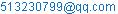(Qiyu Li)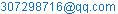(Yixiao Qin)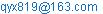(Yixiao Qin)(Yixiao Qin)

*Corresponding author

Qiyu Li, Yixiao Qin, Ming Yang. Stability of Crane’s Lifting Drum with Euler Distribution Forces. International Journal of Mechanical Engineering and Applications. Vol. 4, No. 5, 2016, pp. 176-181. doi: 10.11648/j.ijmea.20160405.12

Received: August 4, 2016; Accepted: August 26, 2016; Published: September 21, 2016

Abstract: In order to optimize structure parameters of lifting drum, the buckling stability of drum on crane is analyzed infinite element method with Euler distribution force which is more appropriate to the realistic load state. The results of finite element analysis are compared with the computing results of traditional simplified method. The research provides a new idea for the stability optimization design on lifting drum as cylindrical shell structure with complex inner rib plate.

Keywords: Lifting Drum on Crane, Buckling Stability, Finite Element Analysis, Euler Distribution Force

1. Introduction

The drum, as the most important force component of crane, the design of stability of it is directly related to the normal work of crane. At present, many important works in this field have been studied, Yixiao Qin represented a coupling between the optimum method of advance and retreat and the finite element analysis for the design on large hoist drums of crane , the finite element analysis of crane’s drum was studied , the buckling stability analysis of 1600t crane’s drum is analyzed based on ANSYS .

Besides, many stability analysis consider the drum as an ideal model of cylinder shell in uniform compression [4, 5, 6]. However, the distribution of stress of drum is mainly concentrated in several circles around it in the real situation. The researches [4,5,6] didn’t respect the characteristics of drum properly or even consider the benefit of complex inner rib plate of drum. Nor did they study the possibility of the nonlinear buckling with drum.

The model of drum is set up based on the feature of structure and stress which depend on the force distribution and equilibrium relationship. The relationship between structural distortion, the variation of stresses and wall thickness of crane are studied, then the results between FEM and conventional design method based on experience design and static design are compared. The coefficients of eigenvalue stability and several unstable modes of drum come out. Finally, the heavy and structure parameters of crane’s drum are optimized for the model is imitated properly.

Normally, the braking of heavy weight decline is considered the most dangerous work situation, as shown in Fig. 1.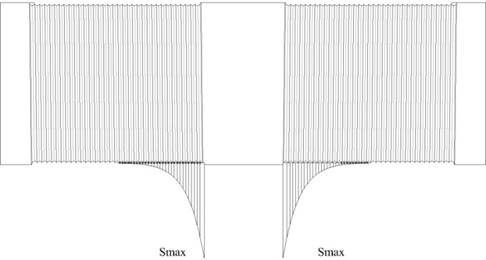Fig. 1. Single rope drum stress distribution.

Most researches assume that the screw ropes are seal off and the identical stress is spread in groove well-distributed [7, 8]. The drum is regarded as axisymmetrical for overlooking the rope’s axis force to end plate. However, the stress of rope declined from drum contact point along the rope groove gradually, which obeys Euler distribution, the equation is: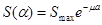(1)

The diameter stress (print to the centre of curvature) is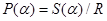the rub force is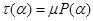distributed along the rope groove for the rope clash the drum.

The direction of rub force can be composed into along the drum and print to drum. Because of the angle between rope groove and drum’s axis is θ, the axis part force is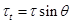the diameter part force is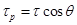.

The tangential friction force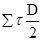equals to the torqueloaded by weight suspension, and the axial force is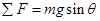.

The horizontal resultant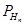and the vertical resultant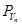are shown as follows: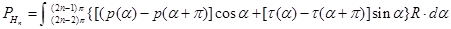(2)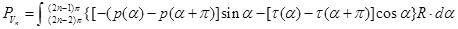(3)

The resultant horizontal moment and the resultant vertical moment are as follows: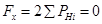(4)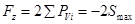(5)

Because the rubbing factor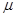of rope and drum is about 0.1 to 0.12 ,

The resultant force of vertical resultant is considered as follow: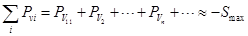(6)

Where minus refers to contrast to the coordinate direction

Then, the torque of drum made by rope is: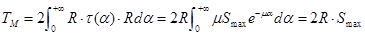(7)

Thus, the twine feature of drum’s rope was ignored for most researchers load the force of rope on drum directly. Although the stress model could reflect the macroscopic force of drum, but the model can’t give accuracy expression to the rope groove’s stress as part of drum’s. As a consequence, the primary model can’t reflect the local buckling of drum for the reason that the primary model does not match the reality.

The stress of rope along the drum changes continuously and obeys the Euler distribution, and the hoop force is proportional to the wire rope tension, as well as the hoop force obeys Euler distribution. Thus, the continuously changed stress must be discretized in FEM. So, the rope stress along groove is loaded on the key points of tiny shell which distributed form rope groove. Every rope groove is divided into N parts, every distributed force is loaded on key points to insure that the join force of rope equals to the join force of key points, as shown in Fig. 2: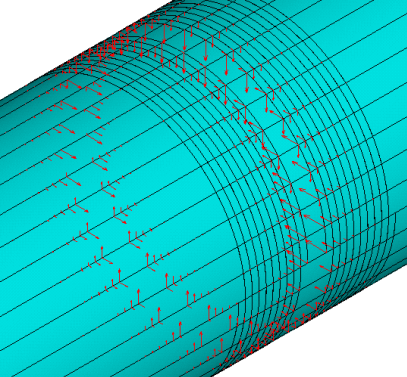3. The Analysis of Eigenvalue Bucking on Drum with Euler Distribution Force

Normally, the buckling stability is divided into the eigenvalue stability and the extreme point of equilibrium and stability. The first one refers that the equilibrium state, internal force and deformation of bucking structural change essentially after buckling. While the second one symbolizes that the key parameters don’t change essentially after buckling. Besides, there is also another buckling stability called jumping bend which doesn’t have the branch point of balance and the extreme point of equilibrium and stability.

Firstly, the eigenvalue stability is analyzed [10, 11].

The critical load of drum is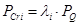(8)

Where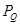refers to the force of rope applied on drum,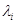refers to eigenvalue of every state.

The equation for eigenvalue of every state is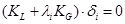(9)

Where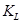is structure stiffness matrix which meets the conditions of small displacement,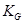is geometric’ stiffness matrices of drum.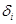is the eigenvectors for I derivative

The first to fourth mode analyzed by ANSYS, as shown in Fig. 3-Fig. 6.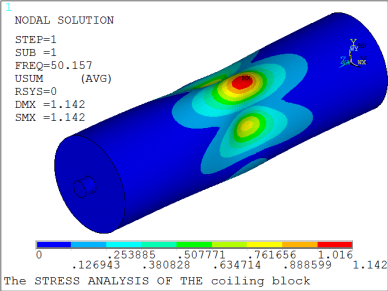Fig. 3. First mode of eigenvalue stability.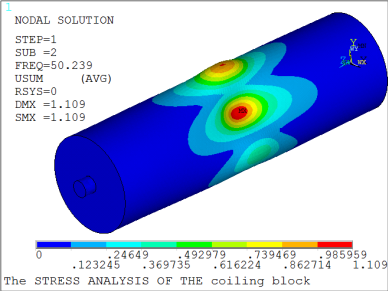Fig. 4. Second mode of eigenvalue stability.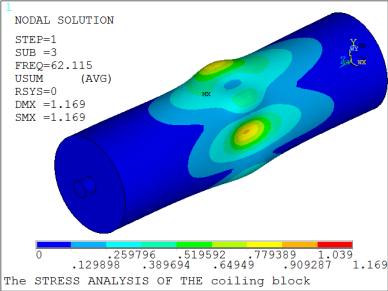Fig. 5. Third mode of eigenvalue stability.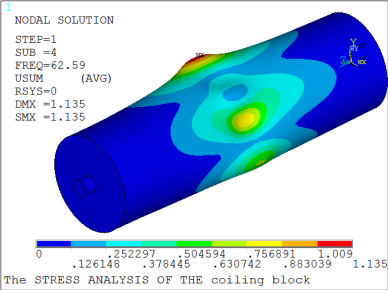Fig. 6. Fourth mode of eigenvalue stability.

As can be seen from the results: 1) when the gripping force reached to the critical pressure, the bucking deformation of the drum took place, the state of instability declined from the middle of drum to the edge of drum, and the drum have feature of radial wave patterns for the griping force loaded on drum. 2) Since the force which loaded on the drum is ultimate strength when having static analysis [12,13], the coefficient of first mode is the stability coefficient, the feature is 50.157, as shown in Fig. 3. Thus, the result shows that the wall thickness of drum is too thick and it is hard to destabilization failure before force reach to buckling.

The stability problem gets highlighted for the decrease of the wall thickness. So, the relationship between the wall thickness of drum and eigenvalue is shown in table 1, and the graph of it is shown in Fig. 7.

Table 1. The coefficients of eigenvalue bucking (CEB) in different thickness of drum.

 Thickness of drum/mm Maxstress/MPa CEB 32 104.589 50.157 30 112.381 45.376 28 120.392 41.039 26 130.428 37.282 24 141.271 33.729 22 150.934 30.404 20 162.223 27.684 18 169.474 24.466 16 189.526 20.918 14 216.154 17.555 12 248.583 14.614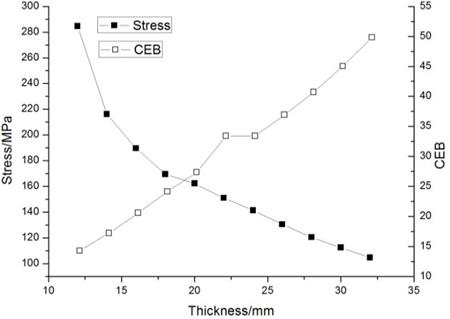Fig. 7. The coefficients of eigenvalue bucking in different thickness of drum.

It is conclusion that with the thickness of cylinder wall becoming more thinness, the stability coefficient of drum decrease. But the critical load is still higher more than 10 times than calculation load, thus, equilibrium bifurcation buckling can hardly happen.

4. The Nonlinear Stability Analysis of Drum with Euler Distribution Force

The nonlinear stability analysis of drum is based on the result of eigenvalue analysis. The large deformation of structure and the sensitivity of the structure defects are considered in the nonlinear stability analysis, thus, the result is more accurate than eigenvalue analysis. Step wise loading way of solving the critical load on the structure of the block is used, and the change process of the structure buckling is tracked at the same time.

The process of nonlinear stability analysis is mainly as follows:

(1) Reasonable incremental step load is set up. In order to obtain accurate critical load value, the dichotomy and automatic time step is used in which case the load increment is small enough when the stress comes near the critical load of drum. As a result, the stability of a large calculation results due to the large step load is avoided.

(2) Automatic time step is set up. The load step is set automatically according to the solving state in ANSYS. The working principle is that, the program would return to step N-1 if the result is misconvergence when it comes to step N, and then halve the load step to calculate again, until the result convergence or load step is small enough.

(3) Initial defects of modify the drum’s structure model is applied. The buckling deformation features which comes form eigenvalue above are used as initial defects to amend the model.

(4) Reasonable solver is chose. The sparse matrix is used to solve this problem, for the reason that it is useful when the model is small or there is ill-conditioned cell in the structure model.

The result of nonlinear stability analysis is shown in Fig. 8.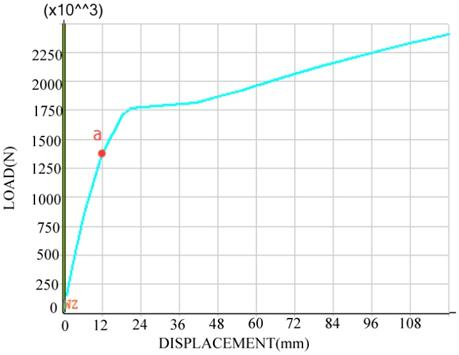Fig. 8. The geometric nonlinear stability analysis results.

The Fig. 8 shows the deformation of drum and rope tension meet small displacement relationship before point a. While the deformation of drum increase obviously with the increase of strain, so that the drum have the ability of resisting critical load when the rope tension exceed point a (1800N), which is so-called the carrying capacity of post-buckling. The table 2 provides some data regarding the critical tension of rope in geometric nonlinear stability is 1.4×106N, decreased by 52.1% than the critical tension of eigenvalue bucking. The graph of the result is shown in Fig. 9.

Table 2.The geometric nonlinear buckling critical tension in different thickness of drum.

 Thickness of drum/mm Critical tension/×105N Decline ratio of nonlinear critical 32 41.5 58.6% 30 36.4 59.9% 28 32.5 60.4% 26 32 57.1% 24 28.4 57.9% 22 23.2 61.8% 20 22.8 58.8% 18 18.2 62.8% 16 15.3 63.4% 14 14.5 58.7% 12 14 52.1%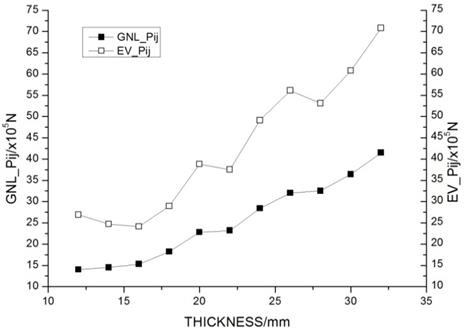Fig. 9. The geometric nonlinear buckling critical tension in different thickness of drum.

The Fig. 9 depicts that as the decline of the wall thickness, the critical tension of drum structure gradually reduce. In short, the result indicates that the influence of wall thickness to the stability of drum is apparent.

5. The Nonlinear Stability Analysis of Drum Considering Material Properties

The large deformation of structure and sensitivity of the structure defects are analyzed above. By the result, the maximum stress far more exceeds than the allowable stress, thus, the material yield before the structure of drum. So, the ultimate bearing capacity of steel is considered. The steel feature is set for Von-Mises yield criterion in ANSYS.

The loading situation of the drum is complex and local deformation is small, thus, the stress train relationship of materials of material reaching the yield limit into plastic state must be defined by defining the enhancing criterion. The multilinear servo reinforcement effect is adopted base on stress-strain relationship curve of steel. The stress-strain relationship is approximated by five segments, as shown in Fig. 10.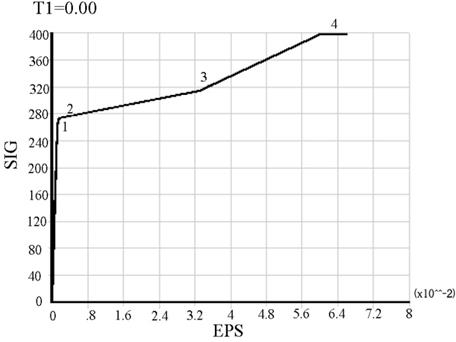Fig. 10. Stress strain curves of Q345.

When the drum comes into plastic deformation condition based on follow-up reinforcement principle, the so called Bauschinger effect, compression yield strength decrease due to the tensile yield strength increase, obey the Mises yield criterion of small strain of metal plastic deformation.

Then, the iterative process of multi linear kinematic analysis of drum is shown in Fig. 11.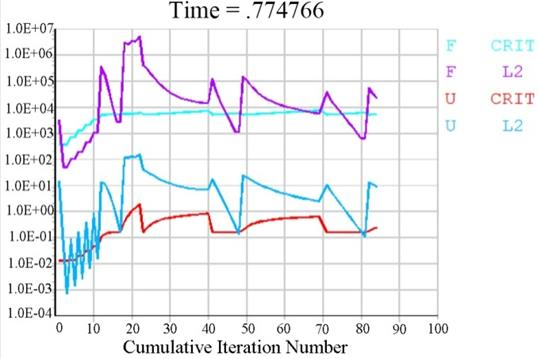Fig. 11. The iterative process of multi linear kinematic analysis.

It can be obtained that the elastic limit of material is 261MPa then the rope tension reached point a, the drum displacement and rope tension basically met small displacement linear relationship before point a, as is shown in Fig. 10. The material of drum came into plastic deformation range and the displacement increases obviously when the rope tension exceed point a. The stress diagram is shown in Fig. 12. The geometric nonlinear characteristic of drum is caused by the increase of deformation. Thus, the solving speed is very slow for the reason that the elastic stiffness matrix and geometric stiffness matrix must be corrected in next iteration.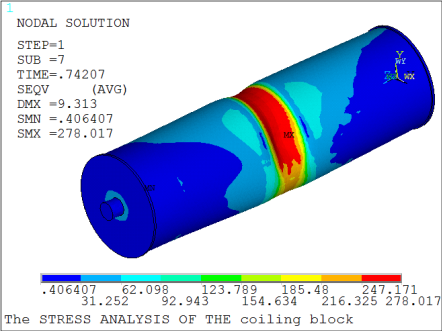Fig. 12. The stress diagram of the material enters the plastic state.

6. Conclusion

The bearing performance of stability of crane’s drum is studied based on the force model obeys Euler distribution. Some of instability models and coefficients of stability are reached by searching eigenvalue buckling of different thicknesses of drum. The maximum allowable stress of rope of buckling drum is confirmed by analyzing the stability of nonlinear large deformation with force model obeys Euler distribution stress and tracking the displacement of rope’s contact point. Thus, the buckling coefficient of drum to the nonlinear instability is determined. Refer to the result of chapter four, this method is an accuracy method which close to the real stress state and can be used to design crane drums precisely.

Acknowledgements

This work was supported by the Natural Science Foundation of Shanxi Province, China (Grantno. 2013011022-6)

References

1. Yixiao Qin, Yuming Cheng, Structure Analysis and Optimun for Hoist Drum with Inner Ribs Bearing Complex External Force, China Mechanical Engineering. (2006) 17 (9): 885-888.
2. Jiankang Luo, Bo Yin, Finite Element Analysis and Structural Optimization of Crane’s Drum, Machinery Design & Manufacture. (2010) 11 (11).
3. Xuejun Hu, Qigui Zhang, 1600t Crane Welding Drum of Buckling Analysis, Construction Machinery. (2013) 8: 97-99.
4. Minggang Zhou, Yong Wang, Qibai Huang, Study on the stability of drum brake non-linear low frequency vibration model, Arch Appl Mach. 77 (2007): 473-483.
5. Y. F. Ding, W. Fang, D. G. Wang, B. Y. Sheng, The Strength Analysis of the Turbine Blade Based on Finite Element Method Calculation and Optimization, Applied Mechanics and Materials. (2014), 9: 549-554.
6. Sun Wei min, Tong Ming bo, Dong Dengke, etal. Post-buckling and stability studies of curved stiffened panels subjected to an axial compression load. Journal of Experimental Mechanics, (2008), 23 (4): 333-338.
7. Achchhe L, Jagtap KR, Singh BN. Post buckling response of functionally graded materials plate subjected to mechanical and thermal loadings with random material properties. Applied Mathematical Modeling, (2013), 37 (5): 2900-2920.
8. Yumin Cheng. The complex variable meshless method for nonlinear problems. In: Frontiers in Applied Mechanics, Imperial College Press, (2014).
9. Peifa Xue Calculating Method of Winding Force Strip-Winding Cylinder Taking Account of Bending Stress, Machine Design (1986).
10. Jun Shu, Chao Xu, Eigen value Buckling Analysis of Drum on Crane Based on ANSYS, Design & Calculation. (2014). 7.
11. Schenk CA, Schueller GI. Buckling analysis of cylindrical shells with random geometric imperfections. International Journal of Non-linear Mechanics, (2003), 38 (7): 1119-1132.
12. Guang-zheng Wang, Zhi-gang Li Compressive Deformation Analysis on New Plate and Airbag Composite Structures, Engineering Mechanics. (2016). 7.
13. QiuZhao, Zhansheng Zhai Analysis of Buckling Modes and Critical Buckling Stress of Open-rib Stiffened Plate, Journal of Architecture and Civil Engineering. (2016) 33 (2).

 Contents 1. 2. 3. 4. 5. 6.
Article ToolsAbstractPDF(513K)